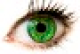#A network for students interested in evidence-based health care

# Hypothesis Testing

Posted on August 1, 2014 by Ashline Amilcar

Tutorials and FundamentalsMost of the time in a research project, it is not economically feasible to work with an entire population. It is preferable to select an adequate sample and statistically infer characteristics of the population. One of the most used methods to make statistical inference is the hypothesis testing: a hypothesis testing requires establishing a null hypothesis (Ho) that is assumed to be true and an alternative hypothesis (Ha) that contradicts the null. The alternative hypothesis represents what we would expect if the null hypothesis were false.

Example:

• Hypothesis: “Value of the systolic blood pressure(SBP) of the population is 120mmHg on average”.
• Ho: “The mean of systolic blood pressure of the population is 120mmHg” (Ho: SBP=120mmHg)
• *Ha: The mean of systolic blood pressure of the population is not 120mmHg (Ha: SBP ≠120mmg)

*Notice when we state that, in the alternative hypothesis, the SBP is different from 120 mmHg we also mean that it can be greater than 120 mmHg or lesser.

In the example above after performing the experiment, suppose that the result of the mean of the SBP is greater than 120mmHg, what is the probability that we obtain this result  because we selected by chance only participants with high SBP values? In other words we can´t automatically conclude from the sample that the population´s SBP is 120mmHg on average just because we have reached this conclusion in the sample; not until we test the hypothesis that the average in the sample is the same as the average in the population.

When performing a hypothesis test to be able to make inference, we assume that Ho is true. If we conclude wrongly that it is false and reject it, we make an error (type I error). The significance level (α) is the probability that this type I error has occurred. The maximum type I error commonly allowed is 5%; 1% significance level and 0.1% are also used when a high grade of certainty is needed.

Most statistical tests calculates a p-value to be compared with an established significance level. P-value reflects, assuming that Ho is true, how well data in the sample are consistent with the Ho. Small p-values provide high evidence against the Ho and indicate that it is very unlikely that the obtained result from the sample is due to chance. If p-value is smaller than the established significance level, the null hypothesis is rejected and we accept the alternative. The result of the research is usually called statistically significant for a p-value  <0.05.

Suppose that the p-value is <0.001 for the above example, If we repeat the experiment several times, the probability that we observe SBP= 120mmHg more times is very low, Ho is rejected in this case and Ha becomes a better option for conclusion.

## 2 Comments on Hypothesis Testing

•Judy Hante

Hi Ashline, In this statement “The result of the research is usually called statistically significant for a p-value >0.05.” do you mean <0.05 ? Thanks, this is a nice summary for understanding tests of hypothesis in journal articles.

September 23, 2014 at 12:51 am
Reply to Judy
•Ashline

Hi Judy. I´m glad that the post helped you, thanks for comment. You are absolutely right, the correct sentence is “The result of the research is usually called statistically significant for a p-value <0.05". I apologize for the error, I will get it corrected soon.

September 25, 2014 at 11:17 pm
Reply to Ashline